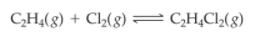×
Get Full Access to Introductory Chemistry - 5 Edition - Chapter 15 - Problem 95p
Get Full Access to Introductory Chemistry - 5 Edition - Chapter 15 - Problem 95p

×

# Solved: This reaction is exothermic. If you were a chemist trying to maximize the amountISBN: 9780321910295 34

## Solution for problem 95P Chapter 15

Introductory Chemistry | 5th Edition

• Textbook Solutions
• 2901 Step-by-step solutions solved by professors and subject experts
• Get 24/7 help from StudySoup virtual teaching assistantsIntroductory Chemistry | 5th Edition

4 5 1 330 Reviews
25
1
Problem 95P

This reaction is exothermic.If you were a chemist trying to maximize the amount of C2H4Cl2 produced, which of the following might you try?

Assume that the reaction mixture reaches equilibrium.

(a) increasing the reaction volume

(b) removing C2H4Cl2 from the reaction mixture as it

forms

(c) lowering the reaction temperature

Step-by-Step Solution:
Step 1 of 3

This reaction is exothermic.a) increasing the reaction volume

Increasing volume would increase the amount of reactants and therefore increase product yield due to increasing the number of reactant particles that would be able to react with each other.

b)removing C2H4Cl2 from the reaction mixture as it forms

Removal would prevent chance of the product being broken back down into the reactants.

c)lowering the reaction temperature

Lower the reaction if the reaction is exothermic.according to le chatelier's principle.

Adding Cl2 would have same effect as A, in that would add more reactants so increase reaction rate.

Step 2 of 3

Step 3 of 3

##### ISBN: 9780321910295

Unlock Textbook Solution